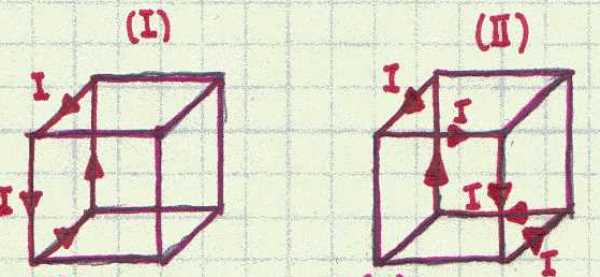# Necker Cube

A constant current $I$ flows around the edges of a cube in two different ways as shown in the figure below. The magnetic induction vectors at the center of the cube in Fig. I and Fig. II are $\vec{B}_{\text{I}}$ and $\vec{B}_{\text{II}}$, respectively. Find the angle in degrees between these vectors.×